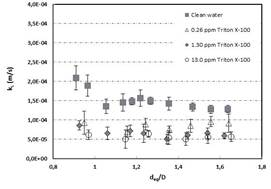# Taylor bubble non-interface data

## 1. Circular channel

### 1.1 Sherwood number

The modified Sherwood numbers versus the ratio of equivalent bubble diameter over channel diameter deq/D are shown in Fig. 1a, b for the 6mm and 8 mm channel, respectively .

 (a)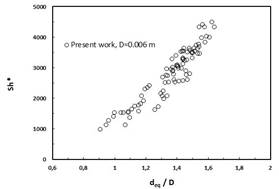(b)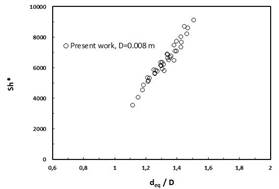Fig. 1 The modified Sherwood numbers versus the ratio of equivalent bubble diameter and channel diameter in 6 mm channel (a) and 8 mm channel (b) 

### 1.2 Oxygen dissolution

The absorption of oxygen in deionized water was investigated. The experimental set up and conditions are the same as
before. Fig. 2 presents dissolution of O2 long bubbles with different initial size. Despite of high solubility of oxygen in water, the dissolution rate of this component in water is orders of magnitude slower than CO2. Therefore it is necessary to consider counter diffusion of dissolved nitrogen N2 in the water .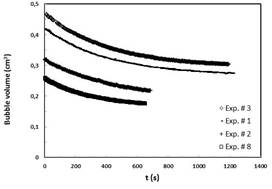Fig. 2 The long term dissolution of O2 bubbles with different initial size

## 2. Square channel

### 2.1 Bubble rise velocity

Fig. 3 shows the measured rise velocity of a freely rising Taylor bubble in circular and square channels as a function of bubble size ratio where deq is sphere-volume equivalent bubble diameter and D is the channel internal diameter. The maximum uncertainty in measured bubble rise velocity is estimated to be 1.09%. The prediction of the correlation proposed by White and Beardmore is also presented in Fig. 3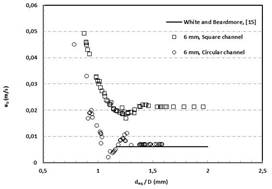Fig. 3 Bubble rise velocity as a function of bubble size ratio

## 2.2 Bubble dissolution

Fig. 4 shows the comparison between the dissolution curve of CO2 Taylor bubbles in circular and square channels. As it can be seen the trend of the dissolution curves of square channels is more even while the dissolution curves of circular channels show some distinguishable changes in the slope .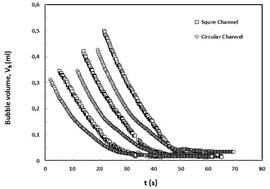Fig.4 Comparison of long term dissolution of CO2 bubbles in circular and square channels

### 2.3 Liquid side mass transfer coefficient

Fig. 5 shows the measured liquid side mass transfer coefficient based on Eq. 11 for stationary Taylor bubbles. The data with the same symbol represents a single bubble over the dissolution period as a function of the instantaneous equivalent diameter and V0 denotes the initial bubble volume .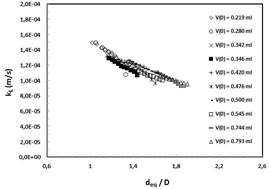Fig. 5 The long term liquid side mass transfer coefficients kL for stagnant single bubbles in square channel

## 3. Presence of surfactant

### 3.1 Bubble rise velocity

The free rise velocity of bubbles in the channel was precisely measured utilizing X-ray radiographic images. The
position of bubbles in a height-range of 30 mm was traced and rise velocity was calculated. The measured data both for clean and contaminated bubbles are shown in Fig. 6 .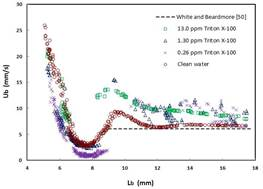Fig. 6 Bubble rise velocity as a function of bubble length in clean and contaminated water

### 3.2 Mass transfer coefficient

The liquid side mass transfer coefficients, kL, according to Eq. (20) for single bubbles in pure and contaminated water are presented in Fig. 7. The values of mass transfer coefficients plotted in this figure are averages of
at least five measurements .